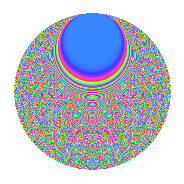# Properties

 Label 2100.2.btLevel 2100 Weight 2 Character orbit bt Rep. character $$\chi_{2100}(239,\cdot)$$ Character field $$\Q(\zeta_{10})$$ Dimension 1440 Sturm bound 960

# Related objects

## Defining parameters

 Level: $$N$$ $$=$$ $$2100 = 2^{2} \cdot 3 \cdot 5^{2} \cdot 7$$ Weight: $$k$$ $$=$$ $$2$$ Character orbit: $$[\chi]$$ $$=$$ 2100.bt (of order $$10$$ and degree $$4$$) Character conductor: $$\operatorname{cond}(\chi)$$ $$=$$ $$300$$ Character field: $$\Q(\zeta_{10})$$ Sturm bound: $$960$$

## Dimensions

The following table gives the dimensions of various subspaces of $$M_{2}(2100, [\chi])$$.

Total New Old
Modular forms 1952 1440 512
Cusp forms 1888 1440 448
Eisenstein series 64 0 64

## Trace form

 $$1440q + O(q^{10})$$ $$1440q - 8q^{10} - 24q^{16} + 28q^{24} + 24q^{30} + 30q^{36} + 80q^{37} + 8q^{40} + 50q^{42} + 24q^{45} + 130q^{48} + 1440q^{49} + 78q^{54} - 60q^{58} - 72q^{60} + 32q^{61} - 24q^{64} - 90q^{66} + 48q^{69} - 12q^{70} + 130q^{78} - 24q^{81} + 56q^{85} - 60q^{88} + 56q^{90} - 148q^{94} - 76q^{96} + O(q^{100})$$

## Decomposition of $$S_{2}^{\mathrm{new}}(2100, [\chi])$$ into newform subspaces

The newforms in this space have not yet been added to the LMFDB.

## Decomposition of $$S_{2}^{\mathrm{old}}(2100, [\chi])$$ into lower level spaces

$$S_{2}^{\mathrm{old}}(2100, [\chi]) \cong$$ $$S_{2}^{\mathrm{new}}(300, [\chi])$$$$^{\oplus 2}$$

## Hecke characteristic polynomials

There are no characteristic polynomials of Hecke operators in the database